# Baumslag-Solitar group

(Redirected from Baumslag–Solitar group)
Jump to: navigation, search

Solitar–Baumslag group

The Baumslag–Solitar groups are a particular class of two-generator one-relator groups which have played a surprisingly useful role in combinatorial and, more recently (the 1990s), geometric group theory. In a number of situations they have provided examples which mark boundaries between different classes of groups and they often provide a testbed for theories and techniques.

These groups have a deceptively simple definition. For each pairandof non-zero integers, there is a corresponding group defined by the presentationwhere, as usual, this notation means that the group is the quotient of the free group on the two generators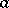and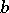by the normal closure (cf. also Normal subgroup) of the single element. When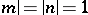,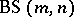is the fundamental group of the torus or Klein bottle (cf. also Klein surface), both of which have been long familiar and well understood — and can therefore be regarded as standing somewhat apart from the remaining groups.

The Baumslag–Solitar groups were introduced in [a1] to provide some simple examples of so-called non-Hopfian groups. A group is called Hopfian (or nowadays Hopf) if every epimorphism from the group to itself is an isomorphism. (The name is derived from the topologist H. Hopf and is thought to reflect the fact that whether fundamental groups of manifolds are "Hopfian" is of interest; cf. also Hopf group; Non-Hopf group.) The condition itself is an instance of what are often referred to as finiteness conditions, i.e. conditions satisfied by finite groups which may or may not hold for infinite groups (cf. also Group with a finiteness condition). It is of interest to see what implications there are among different finiteness conditions within classes of infinite groups.

The main result concerning finiteness conditions and the Baumslag–Solitar groups is as follows:

a)is residually finite (i.e. the intersection of all its subgroups of finite index is trivial) if and only if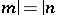or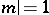or. See also Residually-finite group.

b)is Hopfian if and only if it is residually finite or, where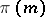denotes the set of prime divisors of. Note that a simple well-known argument shows that a finitely-generated residually-finite group is Hopfian.

Most famously,is non-Hopfian; the mapping: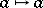,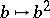, is an epimorphism that is not an isomorphism. It is elementary to show the first (and thatis well-defined) and standard techniques provide an easy verification thatis a non-trivial element of the kernel. An illustration of the weakness of the Hopfian property as a finiteness condition is provided by, which is Hopfian but has a (normal) subgroup of index, the normal closure of, which is non-Hopfian.

(Note that there is a minor error in the actual statement in the original [a1] (which is basically an announcement of results), since it asserts thatis residually finite if one of,divides the other. The observation of this error and its correction are due to S. Meskin [a16].)

The above result has been extended and generalized in various ways, but it appears to be difficult to go beyond results that are somewhat particular and limited in scope.

Although the above theorem divides the Baumslag–Solitar groups into three classes: those that are residually finite, those that are Hopfian but not residually finite and those that are non-Hopfian, the most marked contrast is between those Baumslag–Solitar groups whereor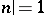and those for which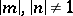.

For a group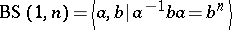there is an obvious homomorphism onto the infinite cyclic group, obtained by setting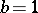, and standard techniques show that the kernel is isomorphic to the additive group of-adic rational numbers. Thus, such groups are meta-Abelian (cf. Meta-Abelian group) and have strong structural properties; in particular, they do not contain a free subgroup of rank two. Moreover, these groups have a particularly simple normal form for their elements: Each element is uniquely represented by a word of the form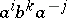where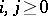and, if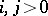,is not divisible by.

When, there is still a strong normal form result since all Baumslag–Solitar groups are examples, indeed are the simplest possible examples, of an HNN-extension (see also [a3] or [a15] for a definition). Hence the following result holds for Baumslag–Solitar groups: Letbe a freely reduced word ofwhich represents the identity element. Thenhas a subword of the form either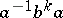where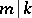, orwhere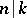.

This result shows, for example, that in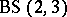the worddoes not represent the identity, and can also be used to show that if, thencontains a free subgroup of rank two.

## Baumslag–Solitar groups as examples and counterexamples.

Below, a number of results concerning Baumslag–Solitar groups will illustrate their role as testbeds in combinatorial and geometric group theory.

### Automorphisms.

The group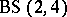, and more generallywhenand one of,divides the other, has an infinitely-generated automorphism group [a4]. A generating set, no finite part of which will generate the automorphism group, consists of the automorphisms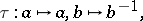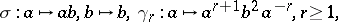plus the inner automorphisms associated toand.

A different kind of failure of finite generation is illustrated by the group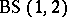, where the automorphism: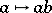,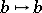has fixed subgroupthat is not finitely generated. This is easily established using the normal form specified above.

### Subgroups.

Groups defined by generators and relations arise from topological and geometric contexts. It is perhaps therefore not altogether surprising that the Baumslag–Solitar groups play a role in questions concerning groups defined by particular topological or geometric conditions. A reasonably classical illustration of this is the fact that only the residually finite Baumslag–Solitar groups can be realized as fundamental groups of "nice"-manifolds (cf. also Three-dimensional manifold; Fundamental group). An exceptionally strong result [a13] shows that those Baumslag–Solitar groups that are not residually finite cannot even occur as subgroups of nice-manifolds, thereby providing a "negativity test" for deciding when a group is a-manifold group.

A contrasting result found in [a14] is that the meta-Abelian Baumslag–Solitar groups are the only finitely-generated (non-cyclic) solvable subgroups of one-relator groups (cf. also Solvable group). In the torsion-free case (when the single relator is not a proper power), this result is a corollary of the theorem in [a11] that the meta-Abelian Baumslag–Solitar groups are the only finitely-generated solvable groups of cohomological dimension two. A further result [a9], more akin to the exclusion result for-manifold groups, is that, for a torsion-free one-relator group, all maximal Abelian subgroups are malnormal if and only ifexcludes as subgroups both the direct product of a free group of rank two with the infinite cyclic group, and all the meta-Abelian groups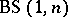,.

### Geometric group theory.

Observations with a flavour similar to that for-manifold groups hold. For example, no Baumslag–Solitar group can be a subgroup of a word hyperbolic group (cf. also Hyperbolic group). For automatic groups, the position is thatis automatic ifbut is otherwise not automatic. It is unknown whether "subgroup exclusion" occurs whenever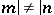. A variant condition, weaker than straight automaticity and known as "asynchronous automaticity" is, however, satisfied by all Baumslag–Solitar groups.

An alternative view of this area is provided by the concept of isoperimetric inequality. This concerns, for a given group presentation, the relationship between the length of an otherwise arbitrary word which represents the identity element and the number of relators required to express this fact. The relationship is linear if and only if the group is word hyperbolic, and for automatic groups it is quadratic in the sense that the number of relators needed is no more than a quadratic function of the length of the word. It is not known (as of 2000) whether the converse to this last statement is true or false, the Baumslag–Solitar groups having, on this occasion, failed to play their traditional role of providing discriminating examples by requiring a number of relators that can always be exponential in the length of the word. (See [a10].)

### Normal forms.

The study of normal forms for elements of a group goes back to the roots of combinatorial group theory and Dehn's introduction of the word problem (cf. also Identity problem). Two ideas relating to normal forms are of interest here. One is that of describing, in terms of the concept of a regular language (cf. also Formal languages and automata; Grammar, regular) properties of particular sets of normal forms and how effectively they can be computed; it was the essential impetus for the study of automatic groups. The second is the idea of a growth function for a group, which is the complex power series whoseth coefficient is the number of elements of (minimal) lengthrelative to some fixed generating set (cf. also Polynomial and exponential growth in groups and algebras). All automatic groups have a growth function which is rational, see [a5], while the residually-finite Baumslag–Solitar groups have rational growth [a2], [a6] but, as noted earlier, are not in general automatic. Interestingly, the argument in [a2] establishing this fact uses finite-state automata, although it is actually the case [a12] that there is no regular set of length-minimal normal forms for the meta-Abelian Baumslag–Solitar groups.

### Rigidity and convexity.

Baumslag–Solitar groups continue to be used as test beds for theories and techniques, in particular those derived from metric space concepts applied to the word metric for a group.

Two illustrations are as follows:

1) An important classification tool for metric spaces is the concept of quasi-isometry: Two metric spaces are quasi-isometric if there are bijective mappings between them in which distortion of distances is uniformly linearly bounded (cf. also Quasi-isometric spaces). A considerable amount of successful classification has been done on groups which arise in specific geometric and topological settings and also on groups which have a nilpotent subgroup of finite index (cf. also Nilpotent group). It has been shown [a7], [a8] that for the meta-Abelian Baumslag–Solitar groups, the position is essentially rigid; namely that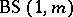andare quasi-isometric if and only if they have isomorphic subgroups of finite index. Furthermore, ifis quasi-isometric to some, then, ignoring a finite normal subgroup,has an index-one or -two subgroupquasi-isometric to. This work is of particular interest since the meta-Abelian Baumslag–Solitar groups do not have a natural geometric setting nor do they satisfy any kind of nilpotency condition.

2) Another metric space concept that has applications to groups is that of "almost convexity" : A presentation of a groupis almost convex if, whenever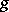andlie within a specific distanceof the origin and are at most a given distance apart, then there is a path of uniformly bounded length which strays no further than distancefrom the origin. The motivation is that almost convexity implies efficient computation of the Cayley graph and this has been studied with successful but not always positive outcomes for the fundamental groups of closed-manifold groups with uniform geometries. In its standard presentation,the meta-Abelian group,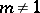, is not almost convex [a18].

## Further reading.

General reference texts in combinatorial and geometric group theory, such as [a3], [a5], [a15], [a17], provide background reading for non-specialists.